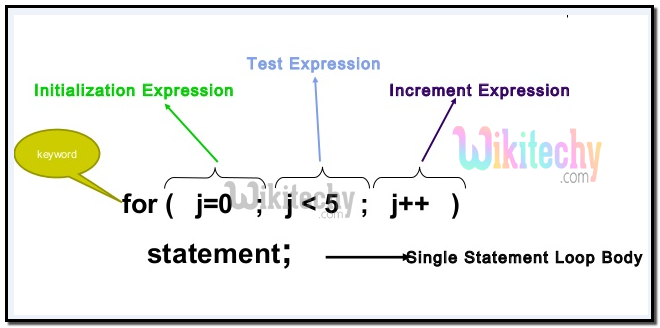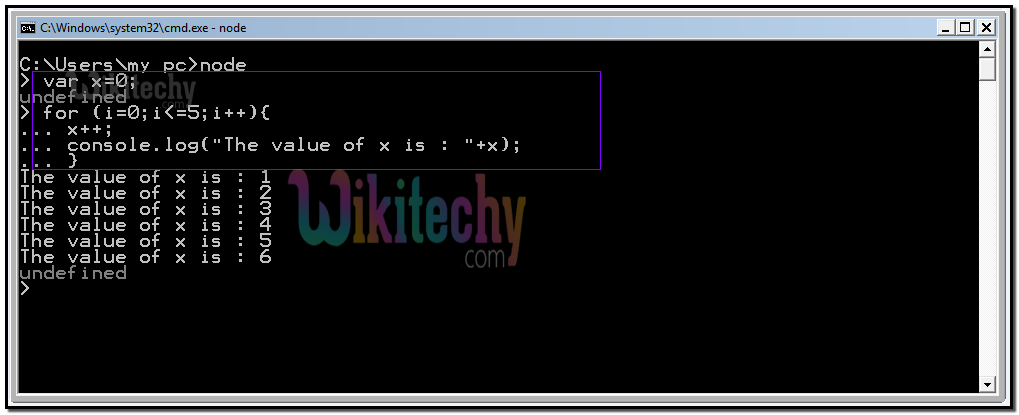# Node js - Loops - nodejs - node js tutorial - node

## What is nodejs loops?

• nodejs loop Provides a controlled looping of data.
• Is one of the familiar programming language control statements.
• Specify iteration of code.
• Allows the code to be executed repeatedly.
• Is often associated with loop variable to allow the body of loop to know about the sequencing.
• Is used in where the number of iterations is predefined without any condition.

## The traditional for-loop got 3 parts :

• 1.the initialization,
• 2.the condition, and finally
• 3.the increment part.

## 1.The initialization :

• a.declares the variables with an initial value.
• b.type of a variable should be same for all variables initialized here.

## 2.The condition :

• a.checks out the condition and proceeds on success.
• b.quits the loop if the condition fails.

## 3.The increment :

• a.increase or decrease so the initialized variable.
• b.depends on the condition part to proceed or stop its duty.

## The above scenarios are explained in figLearn Nodejs - Nodejs tutorial - nodejs loop - Nodejs examples - Nodejs programs

## Code Explanation :Learn Nodejs - Nodejs tutorial - nodejs loop code - Nodejs examples - Nodejs programs

• x is declared with a value 0.
• A for loop is stated with i’s initial value that is 0 and with a condition to check whether i<=5 and with an increment statement to increase the value of i.
• The value of x is incremented and the same is sent for output to console with the line of code “console.log("The value of x is : "+x);

## Node JS Output :Learn Nodejs - Nodejs tutorial - nodejs loop output - Nodejs examples - Nodejs programs

• Executing the for loop in the nodejs framework produces the output of the variable x as shown here as: